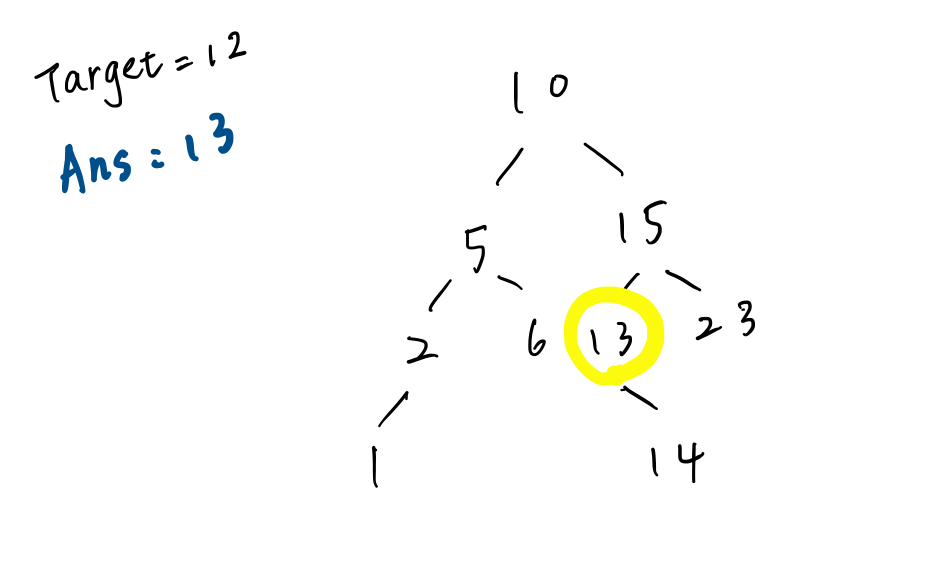#DAY 30
1
Software Development

## Day 30 ：BST中找最接近的值＆感謝文｜infinity - 12(target)｜= infinity
｜10 - 12｜ = 2

｜10 - 12｜= 2
｜15 - 12 ｜= 3

``````function findClosestValueInBst(tree, target) {
return findCloset(tree, target, tree.value);
}

function findCloset(tree, target, closest) {
if (tree === null) return closest;
if (Math.abs(target - closest) > Math.abs(target - tree.value)) {
closest = tree.value;
}
// recursive
if (target < tree.value) {
return findCloset(tree.left, target, closest);
} else if (target > tree.value) {
return findCloset(tree.right, target, closest);
} else {
return closest;
}
}

class BST {
constructor(value) {
this.value = value;
this.left = null;
this.right = null;
}
}

``````
``````function findClosestValueInBst(tree, target) {
return findCloset(tree, target, tree.value);
}
// iterate
function findCloset(tree, target, closest) {
let current = tree;
while (current !== null) {
if (Math.abs(target - closest) > Math.abs(target - current.value)) {
closest = current.value;
}
if (target < current.value) {
current = current.left;
} else if (target > current.value) {
current = current.right;
} else {
break;
}
}
return closest;
}

class BST {
constructor(value) {
this.value = value;
this.left = null;
this.right = null;
}
}

``````### 3 則留言

1

Jen iT邦新手 5 級 ‧ 2021-10-16 00:40:51 檢舉

1

Jen iT邦新手 5 級 ‧ 2021-10-18 19:56:07 檢舉

1

Jen iT邦新手 5 級 ‧ 2021-10-18 19:56:54 檢舉